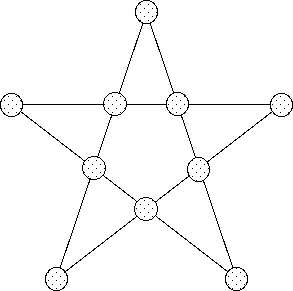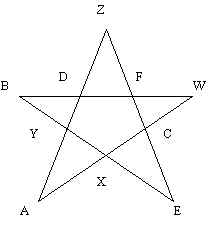Problems & Puzzles: Puzzles

Puzzle 13.- The Prime-magical pentagram

A "pentagram" is a 5 corners star with 5 intersection points as is seen in the following drawing :A "magic pentagram" is any one in which the sum of the 4 points of each of the 5 lines results in the same quantity.

A "prime-magical pentagram" require that all the numbers used in the "magical pentagram" need to be different prime numbers.

Now we are asking for :

The smallest prime-magical pentagram :

1. using (not-necessarily-consecutive) prime numbers
2. using 10 rigorously consecutive prime numbers

(the ‘size’ of the pentagram is defined by the sum of all the 10 numbers used)

<<Note : our (Jaime Ayala and myself) best solutions are kept by G.L. Honaker who has accepted to play in this puzzle as an "honor witness". We will publish our best solutions - let’s say - next October or when somebody else send us better solutions, whichever arrive first>>

Solution

Vasiliy Danilov found (August 03, 1998) that the minimal solution with not necessarily consecutive primes is a pentagram of size 210, being the primes (in ascending order): 5, 7, 11, 13, 17, 19, 23, 31, 41 & 43.

As his result is the same than the one found independently by Ayala & myself,  we can conclude that there is not any better minimal solution.

Vasiliy says that this 10 primes can be located in 120 different ways "including symmetric"

If we represent the pentagram the way Vasiliy does:

A
B C D E
F   G
H
I          J

then this is one of the six solutions sent by him:
A=43, B=13, C=11, D=19, E=41, F=23, G=5, H=31, I=7 and J=17.

Another interesting contribution of Vasiliy is that he has found that every pentagram can be converted as seen in the following figure

A                         G
B C D E              F  A I  C
F   G       <--->    J   E
H                         B
I         J                D       H

interchanging external to internal points

At this point only remains unanswered the second question: "what is the minimal solution with consecutive primes"?

***

Solution to second question:

Harvey Heinz found (October 7, 1998) the same minimal solution than Jaime Ayala and myself, then probably this the absolutely minimal solution. It's formed with the 10 consecutive primes strating with 13907 and ending with 14009.

Our solution is this:A=13907, B=13921, C=13913, D=13933, E=13931, F=13963, X=13997, Y=13967, Z=14009 and W=13999. The sum per line is 55816 and the total sum is 139540.

Soon I'll include the Heinz results.

As a matter of fact, we (Heinz and us) are preparing a combined presentation of our respective methods for solving this puzzle. According to preliminary comments of Heinz we have developed different approaches to this problem.

***

Mr. Robert Stong, from Charlottesville, VA, sent me a letter signed on October 7 which arrived the Monday 19 with the same solution to the second question of the Pentagram puzzle; so he is an independent solver and the third for this puzzle. According to a communication of Mr. Dee Collins, Reference Librarian of the Waynesboro VA, Public Library, Mr. Stong should have gotten his solution before the 1st. of October. I have asked him also for his method.

***Records   |  Conjectures  |  Problems  |  PuzzlesHome | Melancholia | Problems & Puzzles | References | News | Personal Page | Puzzlers | Search | Bulletin Board | Chat | Random Link Copyright © 1999-2012 primepuzzles.net. All rights reserved.